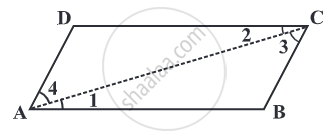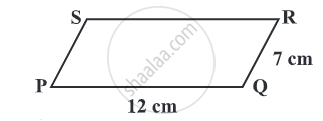# The opposite sides of a parallelogram are of equal length.

Given: ABCD is a parallelogram.To Prove: AB = DC and BC = AD.

Construction: Draw any one diagonal, say bar(AC).

### Proof:

Consider a parallelogram ABCD,

∠ 1 = ∠2, ∠ 3 = ∠ 4             .....(Pair of alternate angle)
and bar(AC) is common side.

Side AC = Side AC              .....(common side)
∠ 1 ≅ ∠2                             .....(Pair of alternate angle)
∠ 3 ≅ ∠ 4                            .....(Pair of alternate angle)

by ASA congruency condition,
∆ ABC ≅ ∆ CDA

This gives AB = DC and BC = AD.

Hence Proved.

#### Example

Find the perimeter of the parallelogram PQRS.In a parallelogram, the opposite sides have the same length.

Therefore, PQ = SR = 12 cm and QR = PS = 7 cm.

So,
Perimeter = PQ + QR + RS + SP
= 12 cm + 7 cm + 12 cm + 7 cm
= 38 cm.
If you would like to contribute notes or other learning material, please submit them using the button below.

### Shaalaa.com

To Prove that the Opposite Sides of a Parallelogram are of Equal length [00:08:44]
S
0%